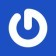# 黑白棋中的 AIPOINT2 Easy()						//人机对战简单AI
{
POINT2 MAX;					//定义以及初始化最优解
MAX.INIT(0, 0);
int maxx = 0;
for (int i = 0; i < SIZE; ++i)
for (int j = 0; j < SIZE; ++j)
{
if (expect[i][j] >= maxx)	//寻找可以转化棋子最多的点作为最优解
{
maxx = expect[i][j];
MAX.INIT(i, j);
}
}
if (ESCEXIT)gameStart();
Sleep(800);					//间歇
return MAX;					//返回最优解
}

 A B C D E F G H 1 90 -60 10 10 10 10 -60 90 2 -60 -80 5 5 5 5 -80 -60 3 10 5 1 1 1 1 5 10 4 10 5 1 1 1 1 5 10 5 10 5 1 1 1 1 5 10 6 10 5 1 1 1 1 5 10 7 -60 -80 5 5 5 5 -80 -60 8 90 -60 10 10 10 10 -60 90AI自己落子某一个位置，得一个正分，之后对手落子某一个位置，所得的分数对于AI来说就是一个负分（即玩家取得的优势，对于AI来说就是劣势）。AI最后的得分：左边是-30分，右边是-15分。最终选择为右边，而不是左边。

int difai(int x,int y,int mapnow[SIZE][SIZE],int expectnow[SIZE][SIZE],int depin,int depinmax)	//极大极小搜索
{
if (depin >= depinmax)return 0;			//递归出口

int maxx = -10005;				//最大权值
POINT2 NOW;
int expectnow2[SIZE][SIZE] , mapnow2[SIZE][SIZE],mapnext[SIZE][SIZE],expectlast[SIZE][SIZE];	//定义临时数组

copymap(mapnow2, mapnow);			//复制当前棋盘

mapnow2[x][y] = NOWCOLOR ? 1 : -1;		//模拟在当前棋盘上下棋
int ME = MAPPOINTCOUNT[x][y] + expectnow[x][y];	//当前棋子权
NOW.INIT(x,y);

Change(NOW, mapnow2, false);			//改变棋盘AI结束

int MAXEXPECT = 0, LINEEXPECT = 0, COUNT = 0;
for (int i = 0; i < SIZE; ++i)
for (int j = 0; j < SIZE; ++j)
{
expectnow2[i][j] = Judge(i, j, !NOWCOLOR, mapnow2);//预判对方是否可以走棋
if (expectnow2[i][j])
{
++MAXEXPECT;
if ((i == 0 && j == 0) || (i == 0 && j == SIZE - 1) || (i == SIZE - 1 && j == SIZE - 1) || (i == SIZE - 1 && j == 0))return -1800;	//如果对方有占角的可能
if ((i < 2 && j < 2) || (i < 2 && SIZE - j - 1 < 2) || (SIZE - 1 - i < 2 && j < 2) || (SIZE - 1 - i < 2 && SIZE - 1 - j < 2))++LINEEXPECT;
}
}
if (LINEEXPECT * 10 > MAXEXPECT * 7)return 1400;//如果对方走到坏点状态较多 剪枝

for (int i = 0; i < SIZE; i++)
for (int j = 0; j < SIZE; j++)
if (expectnow2[i][j])		//如果对方可以走棋
{
int YOU = MAPPOINTCOUNT[i][j] + expectnow2[i][j];//当前权值
copymap(mapnext, mapnow2);	//拷贝地图
mapnext[i][j] = (!NOWCOLOR) ? 1 : -1;		//模拟对方走棋
NOW.INIT(i, j);
Change(NOW, mapnext, false);			//改变棋盘

for (int k = 0; k < SIZE; k++)
for (int l = 0; l < SIZE; l++)
expectlast[k][l] = Judge(k, l, NOWCOLOR, mapnext);	//寻找AI可行点

for (int k = 0; k < SIZE; k++)
for (int l = 0; l < SIZE;l++)
if (expectlast[k][l])
{
int nowm = ME - YOU + difai(k, l, mapnext, expectlast, depin + 1, depinmax);
maxx = maxx < nowm ? nowm : maxx;
}
}
return maxx;
}

### 34 只已被捕捉

•ZGYZSL Chrome | 107.0.0.0 Windows 10

可以参考学习一下源代码？

•千千 Chrome | 107.0.0.0 Windows 10

在这里：https://github.com/im0qianqian/Reversi

•MXang Chrome | 94.0.4606.81 Mac OS X

本科打acm就看千千的blog，现在研究生学计算博弈居然又搜到了哈哈哈哈

•千千 Chrome | 101.0.4951.54 Mac OS X

哈哈哈，学习学习！